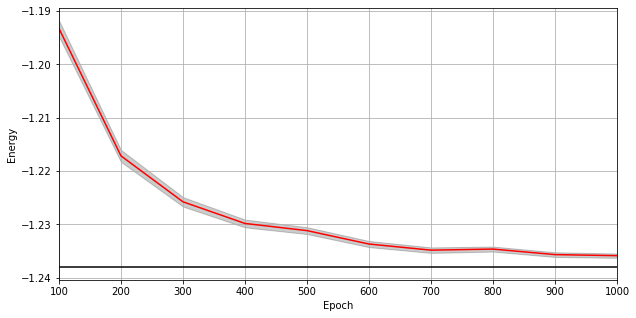This is a static, non-editable tutorial.

We recommend you install QuCumber if you want to run the examples locally. You can then get an archive file containing the examples from the relevant release here. Alternatively, you can launch an interactive online version, though it may be a bit slow:# Training while monitoring observables¶

As seen in the first tutorial that went through reconstructing the wavefunction describing the TFIM with 10 sites at its critical point, the user can evaluate the training in real time with the MetricEvaluator and custom functions. What is most likely more impactful in many cases is to calculate an observable, like the energy, during the training process. This is slightly more computationally involved than using the MetricEvaluator to evaluate functions because observables require that samples be drawn from the RBM.

Luckily, QuCumber also has a module very similar to the MetricEvaluator, but for observables. This is called the ObservableEvaluator. This tutorial uses the ObservableEvaluator to calculate the energy during the training on the TFIM data in the first tutorial. We will use the same training hyperparameters as before.

It is assumed that the user has worked through Tutorial 3 beforehand. Recall that quantum_ising_chain.py contains the TFIMChainEnergy class that inherits from the Observable module. The exact ground-state energy is.

:

import os.path

import numpy as np
import matplotlib.pyplot as plt

from qucumber.nn_states import PositiveWaveFunction
from qucumber.callbacks import ObservableEvaluator

import qucumber
import qucumber.utils.data as data

from quantum_ising_chain import TFIMChainEnergy

# set random seed on cpu but not gpu, since we won't use gpu for this tutorial
qucumber.set_random_seed(1234, cpu=True, gpu=False)

:

train_data = data.load_data(
os.path.join("..", "Tutorial1_TrainPosRealWaveFunction", "tfim1d_data.txt")
)

nv = train_data.shape[-1]
nh = nv

nn_state = PositiveWaveFunction(num_visible=nv, num_hidden=nh, gpu=False)

epochs = 1000
pbs = 100  # pos_batch_size
nbs = 200  # neg_batch_size
lr = 0.01
k = 10

period = 100

h = 1
num_samples = 10000
burn_in = 100
steps = 100

tfim_energy = TFIMChainEnergy(h)


Now, the ObservableEvaluator can be called. The ObservableEvaluator requires the following arguments.

1. period: the frequency of the training evaluators being calculated (e.g. period=200 means that the MetricEvaluator will compute the desired metrics every 200 epochs)

2. A list of Observable objects you would like to reference to evaluate the training (arguments required for generating samples to calculate the observables are keyword arguments placed after the list). The ObservableEvaluator uses a System object (discussed in the previous tutorial) under the hood in order to estimate statistics efficiently.

The following additional arguments are needed to calculate the statistics on the generated samples during training (these are the arguments of the statistics function in the Observable module, minus the nn_state argument; this gets passed in as an argument to fit). For more detail on these arguments, refer to either the previous tutorial or the documentation for Observable.statistics.

• num_samples: the number of samples to generate internally

• num_chains: the number of Markov chains to run in parallel (default = 0)

• burn_in: the number of Gibbs steps to perform before recording any samples (default = 1000)

• steps: the number of Gibbs steps to perform between each sample (default = 1)

The training evaluators can be printed out by setting the verbose keyword argument to True.

:

callbacks = [
ObservableEvaluator(
period,
[tfim_energy],
verbose=True,
num_samples=num_samples,
burn_in=burn_in,
steps=steps,
)
]

nn_state.fit(
train_data,
epochs=epochs,
pos_batch_size=pbs,
neg_batch_size=nbs,
lr=lr,
k=k,
callbacks=callbacks,
)

Epoch: 100
TFIMChainEnergy:
mean: -1.193284     variance: 0.023108      std_error: 0.001520
Epoch: 200
TFIMChainEnergy:
mean: -1.217176     variance: 0.012590      std_error: 0.001122
Epoch: 300
TFIMChainEnergy:
mean: -1.225789     variance: 0.007857      std_error: 0.000886
Epoch: 400
TFIMChainEnergy:
mean: -1.229849     variance: 0.005336      std_error: 0.000730
Epoch: 500
TFIMChainEnergy:
mean: -1.231192     variance: 0.004132      std_error: 0.000643
Epoch: 600
TFIMChainEnergy:
mean: -1.233709     variance: 0.003314      std_error: 0.000576
Epoch: 700
TFIMChainEnergy:
mean: -1.234858     variance: 0.002687      std_error: 0.000518
Epoch: 800
TFIMChainEnergy:
mean: -1.234655     variance: 0.002244      std_error: 0.000474
Epoch: 900
TFIMChainEnergy:
mean: -1.235693     variance: 0.001981      std_error: 0.000445
Epoch: 1000
TFIMChainEnergy:
mean: -1.235892     variance: 0.001680      std_error: 0.000410


The callbacks list returns a list of dictionaries. The mean, standard error and the variance at each epoch can be accessed as follows:

:

# Note that the name of the observable class that the user makes
# must be what comes after callbacks.
energies = callbacks.TFIMChainEnergy.mean

# Alternatively, we can use the usual dictionary/list subscripting
# syntax, which is useful in the case where the observable's name
# contains special characters or spaces
errors = callbacks["TFIMChainEnergy"].std_error
variance = callbacks["TFIMChainEnergy"]["variance"]


A plot of the energy as a function of the training cycle is presented below.

:

epoch = np.arange(period, epochs + 1, period)

E0 = -1.2381

plt.figure(figsize=(10, 5))
ax = plt.axes()
ax.plot(epoch, energies, color="red")
ax.set_xlim(period, epochs)
ax.axhline(E0, color="black")
ax.fill_between(epoch, energies - errors, energies + errors, alpha=0.2, color="black")
ax.set_xlabel("Epoch")
ax.set_ylabel("Energy")
ax.grid()